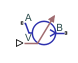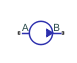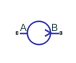# Flow Rate Source (IL)

Generate specified mass flow rate or volumetric flow rate

Since R2020a

•Libraries:
Simscape / Foundation Library / Isothermal Liquid / Sources

## Description

The Flow Rate Source (IL) block represents an ideal mechanical energy source in an isothermal liquid network. The source can maintain the specified mass flow rate or volumetric flow rate regardless of the pressure differential. There is no flow resistance and no heat exchange with the environment. You specify the flow rate type by using the Flow rate type parameter.

Ports A and B represent the source inlet and outlet. The input physical signal at port M or V, depending on the flow rate type, specifies the flow rate. Alternatively, you can specify a fixed flow rate as a block parameter. A positive flow rate causes the fluid to flow from port A to port B.

The block icon changes depending on the values of the Source type and Flow rate type parameters.

Source TypeFlow Rate TypeBlock Icon

`Controlled`

`Mass flow rate``Volumetric flow rate``Constant`

`Mass flow rate``Volumetric flow rate`The block calculates the work performed on the fluid and includes the results in the simulation data log for information purposes:

`${W}_{mech}=\stackrel{˙}{m}\frac{{p}_{B}-{p}_{A}}{\overline{\rho }}$`
`$\overline{\rho }=\frac{{\rho }_{A}+{\rho }_{B}}{2}$`

where:

• Wmech is the mechanical work performed by the source.

• $\stackrel{˙}{m}$ is the mass flow rate generated by the source.

• pA and pB are pressures at ports A and B, respectively.

• $\overline{\rho }$ is the average fluid mixture density.

• ρA and ρB are fluid mixture density values at ports A and B, respectively. Equations used to compute the fluid mixture density depend on the selected isothermal liquid model. For detailed information, see Isothermal Liquid Modeling Options.

If the Flow rate type parameter is set to ```Volumetric flow rate```, the block calculates the mass flow rate from the specified volumetric flow rate:

`$\stackrel{˙}{m}={\rho }_{out}q$`
`${\rho }_{out}=\left\{\begin{array}{ll}{\rho }_{B},\hfill & q>0\hfill \\ {\rho }_{A},\hfill & q\le 0\hfill \end{array}$`

where:

• ρout is the outflow density.

• q is the volumetric flow rate.

For information on viewing logged simulation data, see Data Logging.

### Assumptions and Limitations

• There are no irreversible losses.

• There is no heat exchange with the environment.

## Ports

### Input

expand all

Input physical signal that specifies the mass flow rate through the source.

#### Dependencies

This port is visible if you set the Source type parameter to `Controlled` and Flow rate type parameter to `Mass flow rate`.

Input physical signal that specifies the volumetric flow rate through the source.

#### Dependencies

This port is visible if you set the Source type parameter to `Controlled` and Flow rate type parameter to `Volumetric flow rate`.

### Conserving

expand all

Isothermal liquid conserving port. A positive flow rate causes the fluid to flow from port A to port B.

Isothermal liquid conserving port. A positive flow rate causes the fluid to flow from port A to port B.

## Parameters

expand all

Select whether the flow rate generated by the source can change during simulation:

• `Controlled` — The flow rate is variable, controlled by an input physical signal. Selecting this option exposes the input port M or V, depending on the value of the Flow rate type parameter.

• `Constant` — The flow rate is constant during simulation, specified by a block parameter. Selecting this option enables the Mass flow rate or Volumetric flow rate parameter, depending on the value of the Flow rate type parameter.

Select the type of flow rate, `Mass flow rate` or `Volumetric flow rate`.

Desired mass flow rate of fluid through the source.

#### Dependencies

To enable this parameter, set Source type to `Constant` and Flow rate type to `Mass flow rate`.

Desired volumetric flow rate of fluid through the source.

#### Dependencies

To enable this parameter, set Source type to `Constant` and Flow rate type to `Volumetric flow rate`.

## Version History

Introduced in R2020a